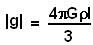## Saturday, September 20, 2008

### Irodov Problem 1.215

From the solution of problem 1.214 we know that the gravitational field vector inside a sphere of uniform density at a point located at radius vector r from the center of the sphere is given by,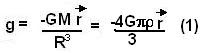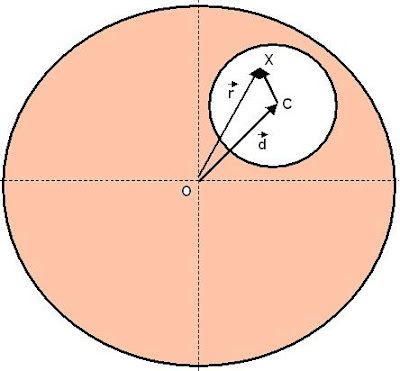Suppose that the center of the spherical cavity, C, is located at a radius vector d relative to the center of the sphere O (as shown in the figure). Since from Gauss law of gravity we know that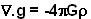The linearity of the equation with respect to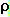indicates that given two or more density distributions we can simply add up the gravitational fields due to each of them to obtain the field due the sum of the densities.

In other words, we can treat the entire hollow sphere as a superposition of two spheres, one a solid sphere of densityand the other a sphere of the exact size and location of the cavity with densityas shown in the figure.

Consider a point X within the cavity located at r from O. The gravitational field strength at X is the sum of the fields due to the two spheres given by,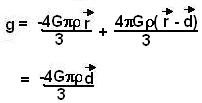This means that the gravitational field will be constant throughout the cavity and directed along the vector d as shown in the figure beside. Since as stated in the problem |d| = l we have,